# Chapter 11 Trigonometric Identities 11 1 Trigonometric Identities

• Slides: 13Chapter 11: Trigonometric Identities 11. 1 Trigonometric Identities 11. 2 Addition and Subtraction Formulas 11. 3 Double-Angle, Half-Angle, and Product-Sum Formulas 11. 4 Inverse Trigonometric Functions 11. 5 Trigonometric Equations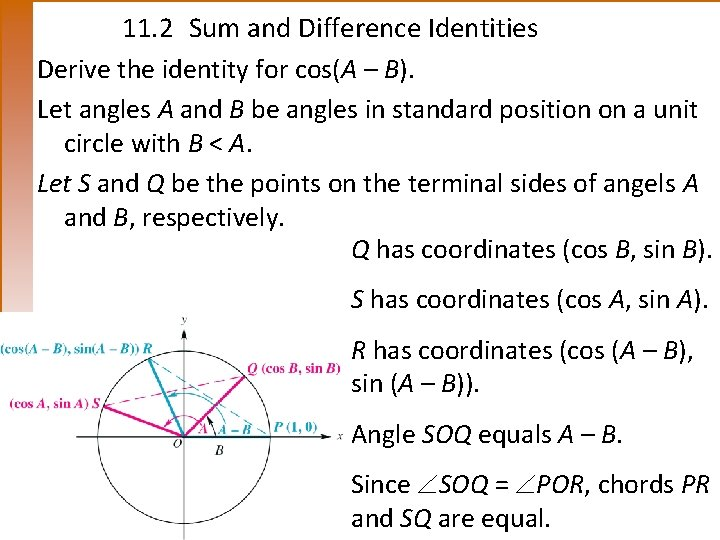11. 2 Sum and Difference Identities Derive the identity for cos(A – B). Let angles A and B be angles in standard position on a unit circle with B < A. Let S and Q be the points on the terminal sides of angels A and B, respectively. Q has coordinates (cos B, sin B). S has coordinates (cos A, sin A). R has coordinates (cos (A – B), sin (A – B)). Angle SOQ equals A – B. Since SOQ = POR, chords PR and SQ are equal.11. 2 Sum and Difference Identities • By the distance formula, chords PR = SQ, Simplifying this equation and using the identity sin² x + cos² x =1, we rewrote the equation as cos(A – B) = cos A cos B + sin A sin B.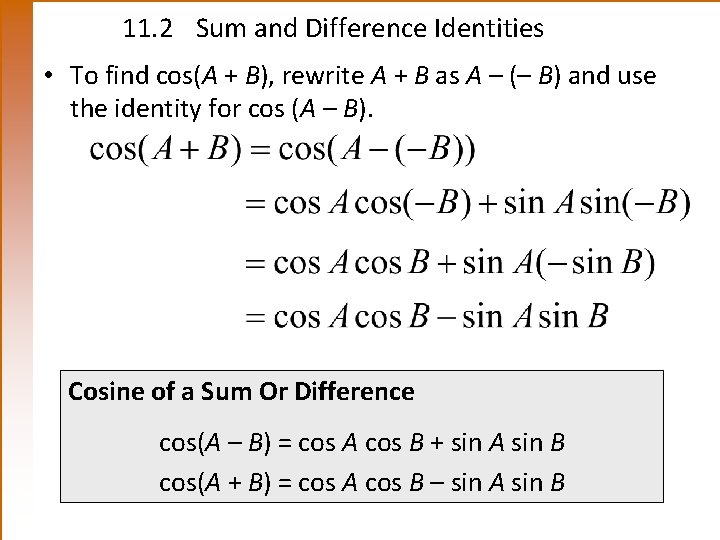11. 2 Sum and Difference Identities • To find cos(A + B), rewrite A + B as A – (– B) and use the identity for cos (A – B). Cosine of a Sum Or Difference cos(A – B) = cos A cos B + sin A sin B cos(A + B) = cos A cos B – sin A sin B11. 2 Finding Exact Cosine Values Example Find the exact value of the following. (a) cos 15° (b)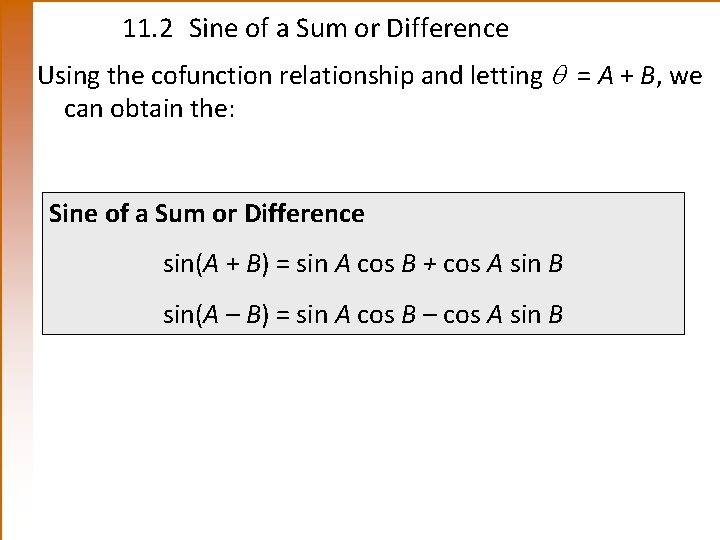11. 2 Sine of a Sum or Difference Using the cofunction relationship and letting = A + B, we can obtain the: Sine of a Sum or Difference sin(A + B) = sin A cos B + cos A sin B sin(A – B) = sin A cos B – cos A sin B11. 2 Tangent of a Sum or Difference • Using the identities for sin(A + B), cos(A + B), and tan(– B) = –tan B, we can derive the identities for the tangent of a sum or difference. Tangent of a Sum or Difference11. 2 Example Using Sine and Tangent Sum or Difference Formulas Example Find the exact value of the following. (a) sin 75° (b) tan (c) sin 40° cos 160° – cos 40° sin 160° Solution (a)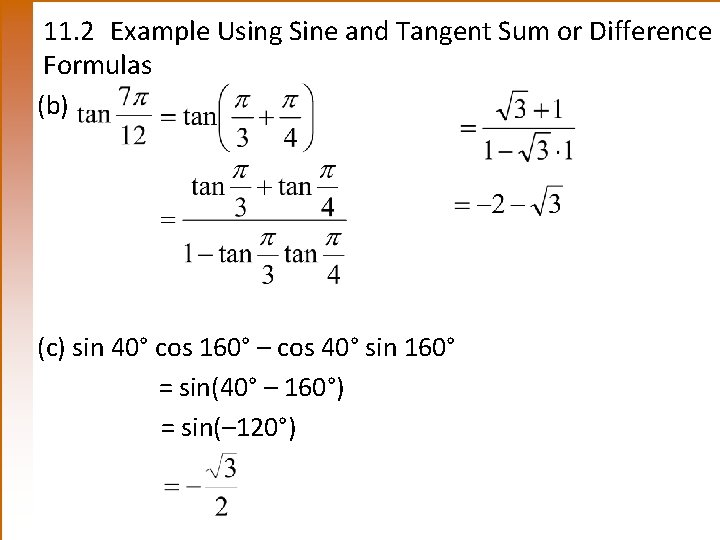11. 2 Example Using Sine and Tangent Sum or Difference Formulas (b) (c) sin 40° cos 160° – cos 40° sin 160° = sin(40° – 160°) = sin(– 120°)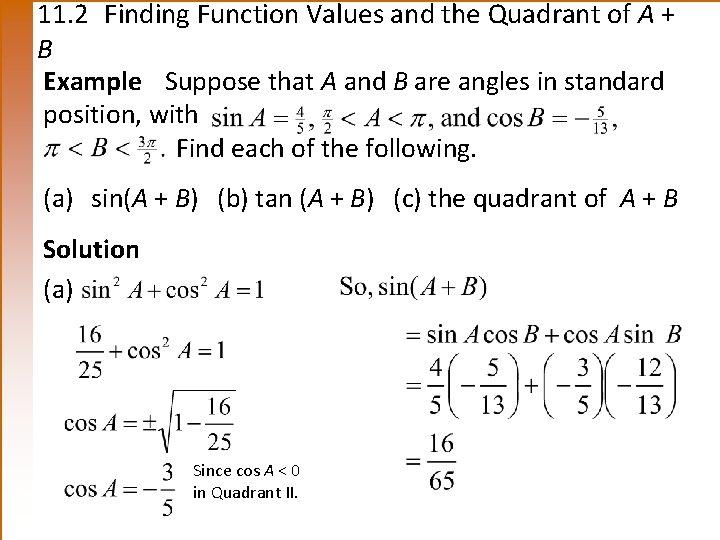11. 2 Finding Function Values and the Quadrant of A + B Example Suppose that A and B are angles in standard position, with Find each of the following. (a) sin(A + B) (b) tan (A + B) (c) the quadrant of A + B Solution (a) Since cos A < 0 in Quadrant II.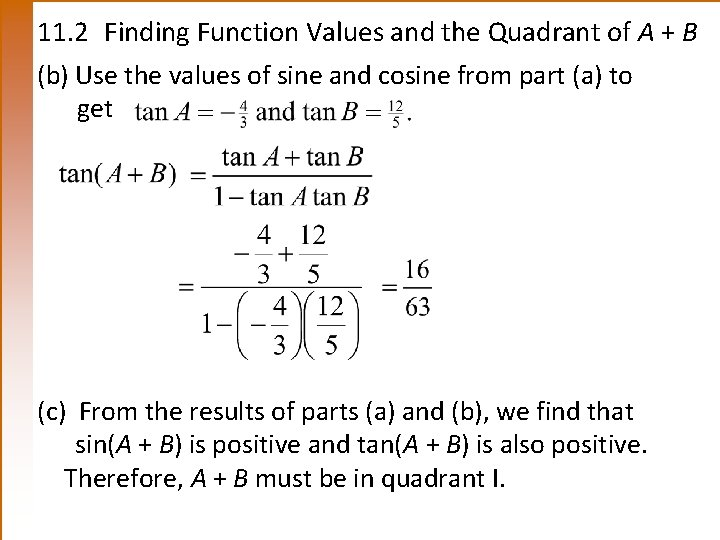11. 2 Finding Function Values and the Quadrant of A + B (b) Use the values of sine and cosine from part (a) to get (c) From the results of parts (a) and (b), we find that sin(A + B) is positive and tan(A + B) is also positive. Therefore, A + B must be in quadrant I.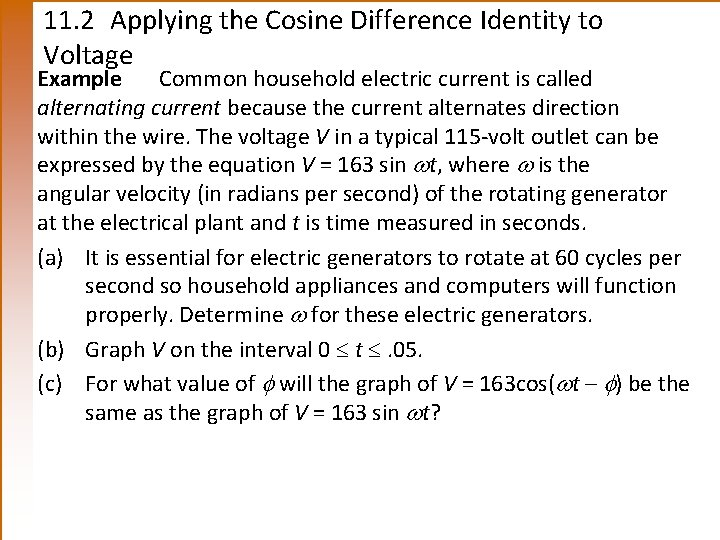11. 2 Applying the Cosine Difference Identity to Voltage Example Common household electric current is called alternating current because the current alternates direction within the wire. The voltage V in a typical 115 -volt outlet can be expressed by the equation V = 163 sin t, where is the angular velocity (in radians per second) of the rotating generator at the electrical plant and t is time measured in seconds. (a) It is essential for electric generators to rotate at 60 cycles per second so household appliances and computers will function properly. Determine for these electric generators. (b) Graph V on the interval 0 t . 05. (c) For what value of will the graph of V = 163 cos( t – ) be the same as the graph of V = 163 sin t?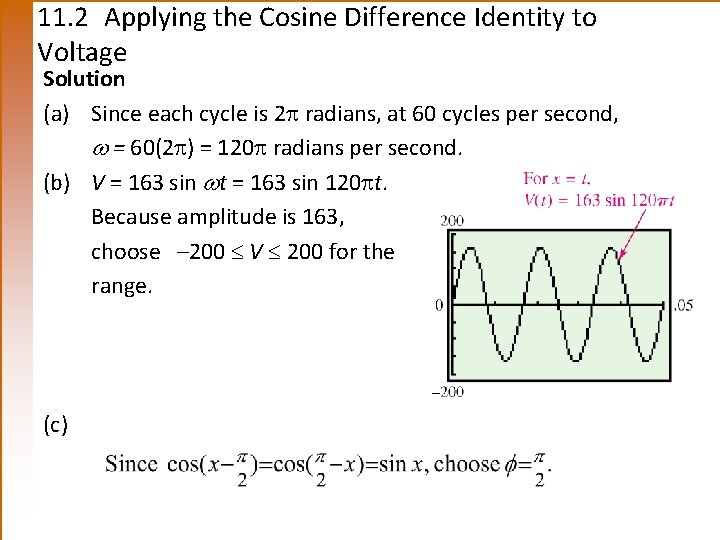11. 2 Applying the Cosine Difference Identity to Voltage Solution (a) Since each cycle is 2 radians, at 60 cycles per second, = 60(2 ) = 120 radians per second. (b) V = 163 sin t = 163 sin 120 t. Because amplitude is 163, choose – 200 V 200 for the range. (c)